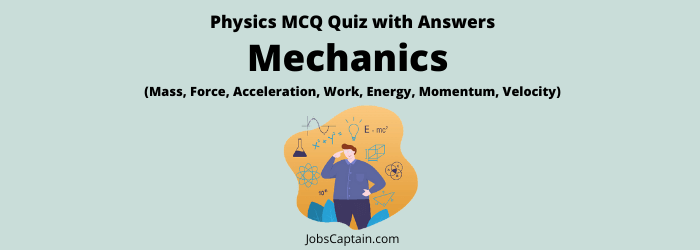# Physics: Mechanics (Mass, Force, Acceleration, Work, Energy, Momentum, Velocity)Question 1. The working principle of a washing machine is _________.

(A) Diffusion

(B) Reverse osmosis

(C) Dialysis

(D) Centrifugation

(D) Centrifugation

Question 2. Which of the following is not a vector quantity?

(A) Volume

(B) Force

(C) Velocity

(D) Displacement

(A) Volume

Question 3. A simple machine helps a person in doing ?

(A) The same amount of work much faster

(B) The same amount of work slowly

(C) The same amount of work with lesser force

(D) Less work

(C) The same amount of work with lesser force

Question 4. A man is standing on a sensitive balance. If he inhales deeply, the reading of the balance ?

(A) May increase or decrease depending on the atmospheric pressure

(B) Remains unaffected

(C) Decreases

(D) Increases

(D) Increases

Question 5. Which of the following is a vector quantity?

(A)  Distance

(B) Displacement

(C) Speed

(D) Time

(B) Displacement

Question 6. Which one of the following is a vector quantity?

(A) Work

(B) Energy

(C) Pressure

(D) Momentum

(D) Momentum

Question 7. Which physical quantity is represented by the ratio of momentum and velocity of the body?

(A) Force

(B) Mass

(C) Acceleration

(D) Velocity

(B) Mass

Question 8. Force is the product of?

(A) Weight and acceleration

(B) Weight and velocity

(C) Mass and acceleration

(D) Mass and velocity

(C) Mass and acceleration

Question 9. If the number representing volume and surface area of a cube are equal, then the length of the edge of the cube in terms of the unit of measurement will be?

(A) 6

(B) 5

(C) 4

(D) 3

(A) 6

Question 10. Energy conservation means?

(A) Energy can neither be created nor destroyed

(B) Energy could not be created but destroyed

(C) Energy could be created

(D) Generation and destruction of energy

(A) Energy can neither be created nor destroyed

Question 11. The energy of wind is _____________.

(A) Potential and kinetic both

(B) Electrical

(C) Only kinetic

(D) Only potential

(C) Only kinetic

Question 12. In wind power, which form of energy is converted into electrical energy?

(B) Solar energy

(C) Potential energy

(D) Kinetic energy

(D) Kinetic energy

Question 13. As the train starts moving, the head of the passenger sitting inside leans backward because of _________.

(A) Conservation of Mass

(B)  Moment of Inertia

(C) Inertia of Motion

(D) Inertia of Rest

(D) Inertia of Rest

Question 14. It is difficult to walk on the ice than on the road because ________.

(A) Ice has lesser friction than the road

(B) Ice does not offer any reaction when we push it with our foot

(C) Road is harder than the ice

(D) Ice is harder than the road

(A) Ice has lesser friction than the road

Question 15. A man jumped at a speed of 5 metre per second from a stationary boat and the boat moved off with the speed of 0.5 metre per second. How many times is the mass of the boat greater than that of the man?

(A)  10 times

(B) 2.5 times

(C) 4.5 times

(D) 5.5 times

(A)  10 times

Question 16. A truck, a car and a motorcycle have equal kinetic energies. If equal, stopping forces are applied and  they stop after travelling a distance of X, Y and Z respectively, then ?

(A) X ≅ 4Y ≅ 8Z

(B) X = Y = Z

(C) X < Y < Z

(D) X > Y > Z

(B) X = Y = Z

Question 17. A person is sitting in a car which is at rest. The reaction from the road at each of the four wheels of the car is R. When the car runs on a straight level road, how will the reaction at either of the front wheels vary?

(A) It shall depend on the material of the road

(B) It will be equal to R

(C) It will be less than R

(D) It will be greater than R

(C) It will be less than R

Question 18. An oil tanker is partially filled with oil and moves forward on a level road with uniform acceleration. The free surface of oil, then ?

(A) assumes parabolic curve

(B) is inclined to the horizontal with larger depth at the rear end

(C) is inclined to the horizontal with smaller depth at the rear end

(D) remains horizontal